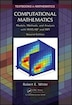# Computational Mathematics: Models, Methods, And Analysis With Matlab And Mpi

## byRobert E. WhiteEditorRobert E. White

November 17, 2015|\$180.50
Hardcover
Earn 903 plum® points
Out of stock online.
Pick up in store
To see if pickup is available,
Find In Store
Not sold in stores
Prices and offers may vary in store

Computational Mathematics: Models, Methods, and Analysis with MATLAB®and MPIis a unique book covering the concepts and techniques at the core of computational science. The author delivers a hands-on introduction to nonlinear, 2D, and 3D models; nonrectangular domains; systems of partial differential equations; and large algebraic problems requiring high-performance computing. The book shows how to apply a model, select a numerical method, implement computer simulations, and assess the ensuing results.

Providing a wealth of MATLAB, Fortran, and C++ code online for download, theSecond Editionof this very popular text:

• Includes a new chapter with two sections on the finite element method, two sections on shallow water waves, and two sections on the driven cavity problem
• Introduces multiprocessor/multicore computers, parallel MATLAB, and message passing interface (MPI) in the chapter on high-performance computing

Computational Mathematics: Models, Methods, and Analysis with MATLAB®and MPI, Second Editionis an ideal textbook for an undergraduate course taught to mathematics, computer science, and engineering students. By using code in practical ways, students take their first steps toward more sophisticated numerical modeling.

Discrete Time¿Space Models Newton Cooling Models Heat Diffusion in a Wire Diffusion in a Wire with Little Insulation Flow and Decay of a Pollutant in a Stream Heat and Mass Transfer in Two Directions Convergence Analysis Steady-State Discrete Models Steady-State and Triangular Solves Heat Diffusion and Gauss Elimination Coolin...